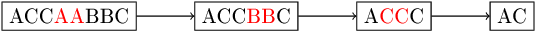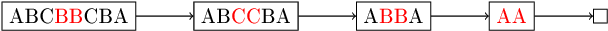hard
Reduce a string containing instances of the letters "A", "B" and "C" via the following rule: remove one occurrence of "AA", "BB" or "CC".
100%
Correctness
100%
Performance
100%

A string S containing only the letters "A", "B" and "C" is given. The string can be transformed by removing one occurrence of "AA", "BB" or "CC".

Transformation of the string is the process of removing letters from it, based on the rules described above. As long as at least one rule can be applied, the process should be repeated. If more than one rule can be used, any one of them could be chosen.

Write a function:

string solution(string &S);

that, given a string S consisting of N characters, returns any string that can result from a sequence of transformations as described above.

For example, given string S = "ACCAABBC" the function may return "AC", because one of the possible sequences of transformations is as follows:Also, given string S = "ABCBBCBA" the function may return "", because one possible sequence of transformations is:Finally, for string S = "BABABA" the function must return "BABABA", because no rules can be applied to string S.

Write an efficient algorithm for the following assumptions:

• the length of string S is within the range [0..50,000];
• string S is made only of the following characters: 'A', 'B' and/or 'C'.